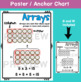# Multiplication Array Worksheets and poster / anchor chartSubject
Resource Type
File Type

PDF

(5 MB|22 pages)
Standards
Also included in:
1. Great resource for extra practice with multiplication arrays practice and multiplication skip counting! ARRAYS PRODUCTWorksheets Include:**Look at the objects in the array and write the multiplication sentence (3 worksheets)**Look at the square arrays in the chart and write the multiplication senten
\$6.75
\$5.00
Save \$1.75
• Product Description
• StandardsNEW

Great resource for extra practice with multiplication arrays practice! Included in this pack are array identifying worksheets, create an array, make arrays and write the problem.

Worksheets Include:

**Look at the objects in the array and write the multiplication sentence (3 worksheets)

**Look at the square arrays in the chart and write the multiplication sentence (3 worksheets)

**Color in an array in the graph from the given problem (3 worksheets)

**Create as many arrays as you can with the objects then write the equations (3 worksheets)

**Create arrays and solve the multiplication sentence pages for x2, x3, x4, x5, x6, x7, x8, x9 (8 worksheets)

20 WORKSHEETS TOTAL

2 POSTERS (black and white and color)

****CHECK OUT THESE SKIP COUNTING POSTERS****

Multiplication Skip Counting Posters

Fluently multiply and divide within 100, using strategies such as the relationship between multiplication and division (e.g., knowing that 8 × 5 = 40, one knows 40 ÷ 5 = 8) or properties of operations. By the end of Grade 3, know from memory all products of two one-digit numbers.
Interpret products of whole numbers, e.g., interpret 5 × 7 as the total number of objects in 5 groups of 7 objects each. For example, describe a context in which a total number of objects can be expressed as 5 × 7.
Total Pages
22 pages
N/A
Teaching Duration
N/A
Report this Resource to TpT
Reported resources will be reviewed by our team. Report this resource to let us know if this resource violates TpT’s content guidelines.